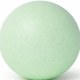Alin - 2 years ago 108
Android Question

# Calculate angle of touched point and rotate it in Android

Math has defeated me once again. This is such a simple task, but I can't manage to get it done.

Scenario: I draw on a SurfaceView a round image. The user touches a point on image border and starts to drag it adround. I need to rotate the circle image according to user movement.
I have two important piece of information, the image center X,Y coordinates and the touched points coordinates.As you can see in the image, the user touched a point, according to my draw the touched point angle should be around 40. I can't manage to calculate it properly.

I tried using this formula:

``````angle = Math.atan2(touchedY - centerY, touchedX - centerX) * 180 / Math.PI
``````

I can't manage to understand how I should calculate the angle, as it is now, it doesn't work properly and values are not good. For instance, in the case of the image, the angle calculate is -50.

LE: Actually I did a mistake I think, as mentioned below. Should the circle be like:vidstige

Let's reformulate the problem: You want to find the angle between two vectors. The first vector is the upvector going straigt up from your center-point (u), and the second vector is the vector from the center point to the touch point (v).

Now we can recall (or google) that

cos a = uv / (|u|*|v|)

Where a is the angle between the vectors and |u| is the length of a vector. The upvector, u, is (0, 1) and has length 1.

Multiplying the vectors by hand cancels the x-term and gives us something like this.

``````double tx = touch_x - center_x, ty = touch_y - center_y;
double t_length = Math.sqrt(tx*tx + ty*ty);
double a = Math.acos(ty / t_length);
``````

Note how the v vector is obtained by subtracting the center point from the touch point. Remember to convert to degrees if needed.

Recommended from our users: Dynamic Network Monitoring from WhatsUp Gold from IPSwitch. Free Download